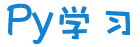注册    登录创作新主题

Python
 python开源   Django   Python   DjangoApp   pycharm
DATA
 docker   Elasticsearch

 问与答   闲聊   招聘   翻译   创业   分享发现   分享创造   求职   区块链   支付之战
aigc
 aigc   chatgpt
WEB开发
 linux   MongoDB   Redis   DATABASE   NGINX   其他Web框架   web工具   zookeeper   tornado   NoSql   Bootstrap   js   peewee   Git   bottle   IE   MQ   Jquery

Python88.com
 反馈   公告   社区推广

Py学习  »  机器学习算法

# 全面总结机器学习超参数调优（附代码）

↑ 点击蓝字 关注极市平台## 三、超参数调优方法

• 网格搜索（Grid Search）：网格搜索是一种简单的超参数调优方法，它通过穷举指定的参数组合，计算每一组参数在验证集上的表现，最终选择表现最好的参数组合。
• 贝叶斯优化：贝叶斯优化是一种利用贝叶斯定理和最优化方法寻找全局最优解的优化算法，它适用于高维、高成本、有限样本的优化问题。
• 随机搜索（Random Search）：随机搜索是一种基于随机采样的超参数调优方法，它通过在参数空间中随机选择参数组合，寻找最优解。

### 3.1 网格搜索Grid Search

1、什么是网格搜索

2、网格搜索的python实战

``from sklearn.model_selection import GridSearchCV  from sklearn.svm import SVC  from sklearn.datasets import load_iris    # 加载数据集  iris = load_iris()  X = iris.data  y = iris.target    # 定义模型  svm = SVC(kernel='linear', C=100, gamma='auto')    # 定义网格搜索参数范围  param_grid = {      'C': [0.1, 1, 10, 100],      'gamma': [1e-3, 1e-2, 1e-1, 1],  }    # 创建网格搜索对象  grid_search = GridSearchCV(svm, param_grid, cv=5)    # 对数据进行网格搜索  grid_search.fit(X, y)    # 输出最佳参数组合和对应的得分  print('Best parameters:', grid_search.best_params_)  print('Best score:', grid_search.best_score_)``
• 使用了Scikit-learn库中的GridSearchCV类来实现网格搜索
• 定义了一个参数网格（param_grid），其中包含了C和gamma两个超参数的不同取值组合
• 创建了一个GridSearchCV对象，并将参数网格、SVM模型和交叉验证（cv）参数传入
• 使用best_params_和best_score_属性输出最佳参数组合和对应的得分

### 3.2 随机搜索Random Search

1、什么是随机搜索

2、基于随机搜索的python实战

``````import numpy as np  from sklearn.datasets import load_iris  from sklearn.ensemble import RandomForestClassifier    # 加载数据集  iris = load_iris()  X = iris.data  y = iris.target    # 定义随机搜索函数  def random_search(X, y, model, param_space, iteration_num):      best_score = -1      best_params = None      for i in range(iteration_num):          # 从参数空间中随机采样一组超参数          params = {k: v[np.random.randint(len(v))] for k, v in param_space.items()}          # 训练模型并计算验证集上的准确率          model.set_params(**params)          score = model.score(X[:100], y[:100])          # 更新最优解          if score > best_score:              best_score = score              best_params = params      return best_score, best_params

# 定义随机森林分类器模型  model = RandomForestClassifier()    # 定义超参数空间  param_space = {      'n_estimators': [100, 200, 300, 400, 500],      'max_depth': [2, 3, 4, 5, 6],      'max_features': ['auto', 'sqrt', 'log2'],      'bootstrap': [True, False]  }    # 执行随机搜索  best_score, best_params = random_search(X, y, model, param_space, 100)  print('最佳准确率：', best_score)  print('最佳超参数：', best_params)``````
• 使用随机森林分类器模型，并定义了四个需要优化的超参数：n_estimators、max_depth、max_features和bootstrap
• 从参数空间中随机采样100组超参数，然后使用验证集上的准确率来评估这些超参数的优劣，最终输出最佳准确率和对应的最佳超参数

### 3.3 贝叶斯优化

1、什么是贝叶斯优化

• 先验函数（Prior Function, PF）：PF主要利用高斯过程回归来建模目标函数的先验分布
• 采集函数（Acquisition Function, AC）：AC主要包括 Expected Improvement（EI）、Probability of Improvement（PI）和 Upper Confidence Bound（UCB）等方法，用于衡量每一个点对目标函数优化的贡献，并选择下一个采样点。

2、贝叶斯优化的python实战

``import numpy as np  from scipy.optimize import minimize  from sklearn.gaussian_process import GaussianProcessRegressor  from sklearn.gaussian_process.kernels import RBF, ConstantKernel as C    # 定义目标函数  def f(x):      return np.sin(5 * x) + np.cos(x)    # 定义高斯过程回归模型  kernel = C(1.0, (1e-3, 1e3)) * RBF(10, (1e-2, 1e2))  gpr = GaussianProcessRegressor(kernel=kernel, n_restarts_optimizer=10)    # 定义贝叶斯优化函数  def bayesian_optimization(X_train, y_train, X_test):      # 训练高斯过程回归模型      gpr.fit(X_train, y_train)      # 计算测试集的预测值和方差      y_pred, sigma = gpr.predict(X_test, return_std=True)      # 计算期望改进（Expected Improvement）      gap = y_pred - f(X_test)      improvement = (gap + np.sqrt(sigma ** 2 + 1e-6) * np.abs(gap).mean()) * 0.5      # 计算高斯过程回归模型的超参数      result = minimize(gpr.kernel_, np.zeros(gpr.kernel_.shape), method='L-BFGS-B')      hyperparameters = result.x      # 输出最优超参数和对应的期望改进值      return hyperparameters, improvement.max()    # 定义贝叶斯优化的迭代次数和采样点数量  n_iter = 20  n_samples = 5    # 进行贝叶斯优化  results = []  for i in range(n_iter):      # 在定义域内随机采样n_samples个点      X_train = np.random.uniform(-2 * np.pi, 2 * np.pi, (n_samples, 1))      y_train = f(X_train)      # 进行贝叶斯优化并记录最优超参数和对应的期望改进值      result = bayesian_optimization(X_train, y_train, X_test=np.random.uniform(-2 * np.pi, 2 * np.pi, (100, 1)))      results.append(result)      print('Iter: {}, Hyperparameters: {:.2f}, Expected Improvement: {:.4f}'.format(i, result, result))``
• 定义目标函数f
• 使用高斯过程回归模型（GPR）来建模目标函数的分布
• 定义贝叶斯优化函数bayesian_optimization；其中训练集、测试集和采样点数作为输入，输出最优的超参数和对应的期望改进值
• Expected Improvement作为采集函数，通过不断地添加样本点来更新目标函数的后验分布，并使用L-BFGS-B方法来最小化高斯过程回归模型的超参数

## 四、基于超参数调优工具

### 4.2 常见的超参数优化工具

• scikit-Opitimize
• Hyperopt
• Optuna
• Spearmint
• Gaussian Process-based Hyperparameter Optimization (GPGO)
• Ray Tune
• GPyOpt
• SigOpt
• Keras Tuner

### 4.3 Scikit-Optimize库

1、Scikit-Optimize库简介

Scikit-optimize 是一个 Python 库，用于执行基于scipy的优化算法。它旨在提供一种简单而有效的工具，用于机器学习和科学计算的优化问题。Scikit-optimize 提供了许多不同的优化算法，包括梯度下降、随机搜索、贝叶斯优化等。

Scikit-optimize 提供了许多预定义的搜索空间和目标函数，以便轻松地设置超参数优化任务。用户可以定义自己的搜索空间和目标函数，以适应特定的机器学习模型和任务。Scikit-optimize 还提供了可视化工具，可以帮助用户更好地理解优化过程和结果。

2、基于python的Scikit-Optimize库优化实战

``import numpy as np  from sklearn.datasets import load_iris  from sklearn.ensemble import RandomForestClassifier  from sklearn.model_selection import train_test_split  from sklearn.metrics import accuracy_score  from skopt import gp_minimize    # 加载数据集  iris = load_iris()  X = iris.data  y = iris.target    # 划分训练集和测试集  X_train, X_test, y_train, y_test = train_test_split(X, y, test_size=0.2, random_state=42)    # 定义机器学习模型  clf = RandomForestClassifier()    # 定义搜索空间和目标函数  search_space = {      'n_estimators': [100, 200, 500],      'max_depth': [3, 5, None],      'max_features': ['auto', 'sqrt', 'log2']  }    def objective(x):      clf.set_params(**{search_space[name]: x[name] for name in x})      clf.fit(X_train, y_train)      y_pred = clf.predict(X_test)      return -accuracy_score(y_test, y_pred)    # 执行优化算法  res = gp_minimize(objective, search_space, n_calls=20, random_state=42)    # 输出最优超参数组合和对应的验证准确率  print("Best hyperparameters:", res.x)  print("Max validation accuracy:", res.fun)``

### 4.4  Hyperopt库

1、Hyperopt库简介

Hyperopt是一个Python库，用于对机器学习模型的算法进行智能搜索。它主要使用三种算法：随机搜索算法、模拟退火算法和TPE（Tree-structured Parzen Estimator）算法。

``pip install hyperopt``

• 准备目标函数：目标函数应该是一个可优化的函数，它接受一个超参数列表作为输入，并返回一个标量值。在Hyperopt中，使用fn来指定目标函数。
• 定义超参数搜索空间：使用Hyperopt的hp模块定义超参数的搜索空间。可以使用hp.choice、hp.uniform等函数来定义不同类型的超参数。
• 使用fmin函数进行优化：使用Hyperopt的fmin函数进行优化，该函数接受目标函数、超参数搜索空间和优化算法作为输入，并返回最佳的超参数组合。

2、基于python优化的实战案例

``from hyperopt import hp, fmin, tpe  from sklearn.datasets import load_iris  from sklearn.model_selection import train_test_split  from sklearn.svm import SVC    # 加载数据集  iris = load_iris()  X = iris.data  y = iris.target    # 划分训练集和测试集  X_train, X_test, y_train, y_test = train_test_split(X, y, test_size=0.2, random_state=42)    # 定义目标函数  def objective(params):      clf = SVC(kernel=params['kernel'], C=params['C'], gamma=params['gamma'])      clf.fit(X_train, y_train)      score = clf.score(X_test, y_test)      return -score  # 因为Hyperopt需要最小化目标函数，所以我们需要将准确率取反    # 定义超参数搜索空间  space = {      'kernel': hp.choice('kernel', ['linear', 'poly', 'rbf', 'sigmoid']),      'C': hp.uniform('C', 0.001, 10),      'gamma': hp.uniform('gamma', 0.001, 10)  }    # 使用贝叶斯优化进行超参数搜索  best = fmin(fn=objective, space=space, algo=tpe.suggest, max_evals=100)  print('Best parameters: ', best)``

### 4.5 Optuna库

1、Optuna库简介

Optuna是一个用于超参数优化的库，它支持定义目标函数，搜索超参数空间并自动进行优化。

``pip install Optuna``

• 定义搜索空间：使用Optuna提供的分布函数来定义超参数的搜索空间。例如，对于一个取值范围为[0, 1]的浮点数，可以使用uniform函数来定义该超参数的搜索空间。
• 定义目标函数：目标函数是需要优化的模型，可以是任何可调用对象，如Python函数、类方法等。目标函数的输入是超参数的值，输出是模型的性能指标。
• 创建Optuna试验：创建Optuna试验对象，并指定目标函数和搜索算法。
• 运行Optuna试验：运行Optuna试验，进行超参数搜索。在每次试验结束后，Optuna会更新超参数的取值，并记录当前试验的性能指标。可以设置尝试的次数或时间，来控制搜索空间的大小和搜索时间的限制。
• 分析试验结果：在试验结束后，可以使用Optuna提供的可视化工具来分析试验结果，并选择最优的超参数组合。

2、基于python的使用案例

``import numpy as np  from sklearn.datasets import make_regression  from sklearn.model_selection import train_test_split  from sklearn.linear_model import SGDRegressor    # 生成数据集  X, y = make_regression(n_samples=100, n_features=1, noise=0.1)  X_train, X_test, y_train, y_test = train_test_split(X, y, test_size=0.2, random_state=42)    # 定义目标函数  def objective(trial):      # 定义超参数      learning_rate = trial.suggest_float('learning_rate', 1e-10, 1e-5)      batch_size = trial.suggest_int('batch_size', 32, 256)      optimizer = trial.suggest_categorical('optimizer', ['sgd', 'adam'])        # 创建模型      model = SGDRegressor(learning_rate=learning_rate, batch_size=batch_size, optimizer=optimizer)        # 训练模型      model.fit(X_train, y_train)        # 计算性能指标      loss = np.mean((model.predict(X_test) - y_test) ** 2)      return loss# 创建Optuna的study对象，并指定需要优化的目标函数和搜索空间import optuna  study = optuna.create_study()  study.optimize(objective, n_trials=100)# 最佳的超参数组合print(study.best_params)  # 输出最佳的超参数组合  print(study.best_value)   # 输出最佳的性能指标值``

### 4.6 Spearmint库

1、Spearmint库简介

Spearmint是一个用于优化贝叶斯推断的库。它基于论文《实用贝叶斯优化》中概述的算法。该库可用于执行贝叶斯优化，这是一种用于全局优化的算法，主要用于寻找最小化目标函数的配置。（建议少使用）

https://github.com/JasperSnoek/spearmint

Spearmint库提供了一种表达和优化贝叶斯推断问题的方式。它允许用户定义目标函数以及用于描述优化问题的约束和边界。库中的核心算法则负责根据这些定义来优化目标函数。Spearmint库的优点包括：

• 灵活的目标函数表达：Spearmint支持多种类型的数据和复杂的模型结构，使得用户可以灵活地表达各种目标函数。
• 高性能：Spearmint通过高效的实现和优化算法来提高运行速度，从而能够处理大规模的数据集和复杂的模型。
• 易于使用：Spearmint提供了简单易用的接口，使得用户可以轻松地配置和运行优化任务。
• 社区支持：Spearmint是由一个活跃的社区支持的开源项目，这意味着用户可以获得来自其他开发者的反馈和支持。

2、基于Spearmint的实战案例

``import numpy as np  import spearmint as sp  from scipy.stats import norm    # 定义目标函数  def objective(x):      # 假设目标函数是一个简单的二次函数，其中x是一个向量      return x**2 + x**2    # 定义Spearmint的优化器  spearmint = sp.Spearmint()    # 设置要优化的参数范围  var_names = ['var1', 'var2']  bounds = [[-5, 5], [-5, 5]]  priors = [sp.priors.NormalPrior(0, 1), sp.priors.NormalPrior(0, 1)]    # 运行贝叶斯优化，设置最大迭代次数为10次  results = spearmint.optimize(objective, var_names, bounds, priors, n_iter=10)    # 输出最优的参数组合和对应的函数值  print('最优参数组合：', results.x)  print('最优函数值：', results.func)``

### 4.7 GPGO库

1、GPGO简介

GPGO全称是Gaussian Process Optimization for Hyperparameters Optimization，是Google的超参数优化库，它使用Gaussian Processes（高斯过程）进行超参数优化，专为TensorFlow和Keras而设计。

``pip install gpgo``

• 首先，需要定义要优化的目标函数（例如，神经网络的训练损失）。
• 其次，需要定义搜索空间，即超参数的可能取值范围。
• 然后，使用GPGO运行优化过程。在每次迭代中，优化器会选择一组超参数，并使用目标函数评估该组超参数的性能。
• 最后，优化器会根据评估结果更新其关于最佳超参数的信念，并继续搜索，直到达到预设的终止条件（例如，达到最大迭代次数或找到满意的超参数组合）。

2、python实战案例

``import numpy as np  import tensorflow as tf  from tensorflow.keras.datasets import mnist  from tensorflow.keras.models import Sequential  from tensorflow.keras.layers import Dense, Flatten  from gpgo import GPGO    # 加载MNIST数据集(x_train, y_train), (x_test, y_test) = mnist.load_data()  # 数据缩放x_train = x_train / 255.0  x_test = x_test / 255.0    # 1-定义神经网络模型  model = Sequential([      Flatten(input_shape=(28, 28)),      Dense(128, activation='relu'),      Dense(64, activation='relu'),      Dense(10)  ])    #2- 定义优化目标函数（即神经网络的训练损失）  def loss_fn(y_true, y_pred):      return tf.reduce_mean(tf.nn.softmax_cross_entropy_with_logits(labels=y_true, logits=y_pred))    # 3-定义优化器并设置超参数的搜索空间  optimizer = tf.keras.optimizers.Adam(learning_rate=1e-4)  hyperparams = {      'kernel__lgcp': [5, 10, 20],      'kernel__scale': [1e-4, 1e-3, 1e-2],      'kernel__lengthscale': [1., 5., 10.]  }    # 4-创建GPGO优化器并运行优化过程  gpgo = GPGO(optimizer=optimizer, loss_fn=loss_fn, hyperparams=hyperparams, datasets=(x_train, y_train), epochs=10)  best_params, best_loss = gpgo.run()``
• 加载MNIST数据集并定义了一个简单的神经网络模型
• 定义了优化目标函数，即神经网络的训练损失
• 创建了一个GPGO优化器，并将超参数的搜索空间传递给它
• 使用`run`方法运行优化过程，并输出最佳超参数和最低的训练损失

### 4.8 Ray Tune库

1、简介

Ray Tune的特性包括：

• 支持多种超参数优化算法，如网格搜索、随机搜索和贝叶斯优化等。
• 可以自动管理实验的进度和结果，提供清晰的可视化界面。
• 可以轻松扩展到分布式环境，支持多节点并行超参数搜索。
• 与Ray框架无缝集成，可以轻松扩展到其他Ray任务中。

2、官网提供的优化实战案例：

``from ray import tunedef objective(config):  # 1-定义目标优化函数    score = config["a"] ** 2 + config["b"]    return {"score": score}search_space = {  # 2-定义超参数搜索空间    "a": tune.grid_search([0.001, 0.01, 0.1, 1.0]),    "b": tune.choice([1, 2, 3]),}tuner = tune.Tuner(objective, param_space=search_space)  # 3-执行搜索过程results = tuner.fit()print(results.get_best_result(metric="score", mode="min").config)  # 最佳超参数组合``

### 4.9 Bayesian Optimization库

1、简介

Bayesian optimization，也称为Bayesian experimental design或sequential design，是一种使用贝叶斯推理和高斯过程（Gaussian process）的全局优化技术。它是一种在尽可能少的迭代次数内找到一个未知函数的最大值或最小值的方法，特别适合优化高成本函数或在勘探和开发之间需要平衡的情况。

``pip install bayesian-optimization``

2、python实战案例

``import numpy as np  from sklearn.gaussian_process import GaussianProcessRegressor  from scipy.optimize import minimize    # 定义目标函数  def target_function(x):      return np.sin(5 * x) + np.cos(2 * x) + np.random.normal(0, 0.1, size=x.shape)    # 定义高斯过程模型  def gpr_model(X, y):      kernel = 1.0 * np.eye(X.shape) + 0.5 * np.ones((X.shape, X.shape))      gpr = GaussianProcessRegressor(kernel=kernel)      gpr.fit(X, y)      return gpr    # 定义Bayesian Optimization函数  def bayesian_optimization(n_iterations, n_initial_points):      # 初始化数据点      x_initial = np.random.uniform(-5, 5, n_initial_points)      y_initial = target_function(x_initial)        # 初始化高斯过程模型      X_train = np.vstack((x_initial, x_initial))      y_train = np.vstack((y_initial, y_initial))      gpr = gpr_model(X_train, y_train)        # 进行n_iterations次迭代      for i in range(n_iterations):          # 使用EI策略选择新的数据点          acquisition_func = gpr.predictive_mean + np.sqrt(gpr.predictive_covariance(x_initial)[:, None, None]) * np.random.randn(*x_initial.shape)          EI = -gpr.negative_log_predictive_density(y_initial, acquisition_func)          x_new = x_initial[np.argmax(EI)]          y_new = target_function(x_new)                  X_train = np.vstack((X_train, x_new))          y_train = np.vstack((y_train, y_new))                 gpr = gpr_model(X_train, y_train)                  x_initial = np.vstack((x_initial, x_new))          y_initial = np.vstack((y_initial, y_new))                 print("Iteration {}: Best value = {} at x = {}".format(i+1, np.min(y_initial), np.argmin(y_initial)))      return x_initial[np.argmin(y_initial)], np.min(y_initial)    # 运行Bayesian Optimization函数并输出结果  best_x, best_y = bayesian_optimization(n_iterations=10, n_initial_points=3)  print("Best x = {}, best y = {}".format(best_x, best_y))``
• 定义了一个目标函数`target_function`，该函数是一个带有随机噪声的正弦和余弦函数的组合；
• 定义了一个高斯过程模型`gpr_model`，该模型使用一个常数核来构建高斯过程；
• 定义了一个`bayesian_optimization`函数，该函数使用贝叶斯优化算法来找到目标函数的最大值；
• 最后调用`bayesian_optimization`函数并输出结果

### 4.10 GPyOpt库

1、简介

GPyOpt是一个基于GPy的Python库，用于实现贝叶斯优化。它提供了灵活的框架，可以处理具有各种类型代理模型（如高斯过程和随机森林）的优化问题。

GPyOpt库旨在解决实际问题，包括但不限于函数优化、超参数优化、深度学习中的模型调参等。使用者可以根据需要自定义代理模型，并且能够方便地与第三方库集成。此外，GPyOpt支持多种优化算法，如贝叶斯优化、粒子群优化等，以满足不同应用场景的需求。

``pip install gpyopt``

``# git clone https://github.com/SheffieldML/GPyOpt.git# cd GPyOpt# git checkout devel# nosetests GPyOpt/testing``

3个主要依赖包的版本要求：

• GPy (>=1.0.8)
• numpy (>=1.7)
• scipy (>=0.16)

2、基于python的使用案例

``import numpy as np  from gpyopt import Optimizer    # 定义目标函数  def f(x):      return -x**2    # 定义初始点  x0 = np.array([0.0])  # 定义优化器  optimizer = Optimizer(f=f, x0=x0)  # 设置优化选项  optimizer.set_verbose(True)  # 设置是否输出优化信息  optimizer.set_stop_condition(stop='max_iter', value=100)  # 设置最大迭代次数和停止条件    # 运行优化  optimizer.optimize()    # 输出结果  print('最优解:', optimizer.x_opt)  print('最优值:', optimizer.f_opt)``

### 4.11 SigOpt库

1、简介SigOpt超参数优化库是一个用于优化机器学习模型的软件库。SigOpt的优化算法使用贝叶斯优化，这是一种用于寻找全局最优的优化算法，通常用于寻找深度学习模型中的最佳超参数组合。

SigOpt的API使得调整模型超参数变得容易，并且可以与许多不同的机器学习库（包括TensorFlow、PyTorch、Scikit-learn等）集成。SigOpt还提供了一个可视化界面，可以帮助用户监控和调整优化过程。通过使用SigOpt，开发人员可以更快地找到最佳超参数组合，提高模型的性能和准确性。

``pip install sigopt  ``

2、基于python的实战案例

``import torch  import torch.nn as nn  from torch.utils.data import DataLoader  from torchvision import datasets, transforms# 优化相关import sigopt  from sigopt.api import create_run  from sigopt.config import get_version_id  from sigopt.local import local_experiment  from sigopt.run import run_fn, get_default_args, get_default_config, get_default_options, get_default_suggestion_callback, run_in_notebook, run_in_script, run_in_jupyter_notebook   # 用于定义运行函数和运行环境  from sigopt.suggestion import get_suggestion, get_suggestion_from_web  # 用于获取建议的超参数值# 定义学习率和批量大小space = {    "learning_rate": (1e-5, 1e-2, "log-uniform"),    "batch_size": (32, 256, "uniform")  }# 定义基于pytorch的深度学习模型class SimpleModel(nn.Module):    def __init__(self):      super(SimpleModel, self).__init__()      self.fc = nn.Linear(784, 10)          def forward(self, x):      x = x.view(-1, 784)      x = self.fc(x)      return x# 加载mnist数据集transform = transforms.Compose([    transforms.ToTensor(),    # 转成张量  transforms.Normalize((0.1307,), (0.3081,))  # 数据标准化  ])  train_dataset = datasets.MNIST(root="data", train=True, transform=transform, download=True)  train_loader = DataLoader(train_dataset, batch_size=128, shuffle=True)# 优化过程def objective(config):  # 定义优化目标函数，即模型的准确率    model = SimpleModel()  # 创建模型实例    optimizer = torch.optim.Adam(model.parameters(), lr=config["learning_rate"])  # 创建优化器并设置学习率    criterion = nn.CrossEntropyLoss()  # 创建损失函数    train_loss = 0.0    correct = 0.0    total = 0.0    for i, data in enumerate(train_loader, 0):  # 对训练集进行迭代，计算损失和准确率      inputs, labels = data  # 获取输入和标签数据      optimizer.zero_grad()  # 清零梯度缓存      outputs = model(inputs)  # 前向传播，计算输出张量      loss = criterion(outputs, labels)  # 计算损失张量      loss.backward()  # 反向传播，计算梯度张量并更新权重参数      train_loss += loss.item()  # 累加损失值并计算平均损失值和准确率         _, predicted = torch.max(outputs.data, 1)  # 找到最大概率的标签作为预测结果并计算准确率      total += labels.size(0)  # 累加样本数以计算总体准确率      correct += predicted.eq(labels.data).cpu().sum()  # 累加正确预测的样本数并计算准确率    accuracy = correct / total  # 计算总体准确率并返回给优化器作为目标函数值    return accuracy, {"learning_rate": config["learning_rate"], "batch_size": config["batch_size"]}  # 将目标函数值和超参数值返回给优化器``

KerasTuner是一个易于使用的分布式超参数优化框架，能够解决执行超参数搜索时的一些痛点。它利用高级搜索和优化方法，如HyperBand搜索和贝叶斯优化，来帮助找到最佳的神经网络超参数。

``pip install keras-tuner --upgrade``

``import keras_tunerfrom tensorflow import keras# 定义网络模型def build_model(hp):    model = keras.Sequential()    model.add(keras.layers.Dense(        hp.Choice("units", [8,16,32]),        activation="relu"    ))    model.add(keras.layers.Dense(1,activation="relu"))    model.compile(loss="mse")    return modeltuner = keras_tuner.RandomSearch(    build_model,    objective="val_loss",    max_trials=5)tuner.search(x_train, y_train, epochs=5, validation_data=(x_val, y_val))``

## 五、基于AutoML库的超参数调优

### 5.1 什么是AutoML库

AutoML的目的是简化机器学习的过程，使非专业人士也可以应用机器学习，或者帮助专业人士更高效地处理机器学习任务。这些库通常提供易于使用的接口，并且能够处理大规模的数据集。

### 5.2 常见的自动化机器学习库

• Auto-Sklearn。Auto-Sklearn是基于scikit-learn软件包构建的开源AutoML库。它为给定的数据集找到最佳性能的模型以及最佳的超参数集。它包括一些特征工程技术，例如单点编码，特征归一化，降维等。该库适用于中小型数据集，不适用大型数据集。
• H2O AutoML。H2O AutoML是一个完整的端到端的机器学习自动化工具，可以处理各种类型的数据集，包括小数据和大数据，标准数据和非标准数据。它实现了整个机器学习流程的自动化，包括数据准备，模型选择，特征选择，模型优化等。
• Auto-Keras。Auto-Keras是一个基于Keras深度学习框架的自动机器学习库。它旨在为深度学习模型提供高度自动化的设计和训练流程，以解决用户在面对不同任务时可能需要重复编写大量代码的问题。
• AutoGluon。AutoGluon是一个开源的深度学习自动机器学习库，由AWS团队开发和维护。它旨在帮助开发者自动完成机器学习的所有过程，包括数据预处理、特征工程、模型选择和超参数调整等。AutoGluon使用了一种称为“神经架构搜索”的技术来自动化地选择最佳的模型架构。
• Pycaret。PyCaret 是一个开源的、低代码的机器学习库，旨在简化机器学习工作流并提高工作效率。它是一个 Python 库，封装了多个流行的机器学习库和框架，如 scikit-learn、XGBoost、LightGBM、CatBoost、spaCy、Optuna、Hyperopt、Ray 等。PyCaret 还提供了一套简单易用的 API，可以帮助你完成各种机器学习任务，包括数据预处理、模型训练、评估和部署等。
• MLBox。MLBox是一个功能强大的自动化机器学习库，旨在为机器学习工程师和研究者提供一站式的机器学习解决方案。它是由国内的一家公司开发的，封装了多种流行的机器学习算法和框架，如scikit-learn、XGBoost、LightGBM、CatBoost、spaCy、Optuna、Hyperopt、Ray等，并将它们整合到一个统一的框架中，提供了简单易用的API，使得机器学习的流程变得更加高效和便捷。
• Auto-PyTorch。Auto-PyTorch是一个基于PyTorch的自动机器学习库。它旨在为超参数搜索和模型选择提供自动化的解决方案，以帮助用户在训练深度学习模型时提高效率和准确性。

### 5.3 Auto-Sklearn

1、简介

Auto-Sklearn是一个开源的AutoML库，它利用流行的Scikit-Learn机器学习库进行数据转换和机器学习算法。Auto-Sklearn是由Matthias Feurer等人开发的。在他们的2015年题为“efficient and robust automated machine learning”的论文中进行了描述。

Auto-Sklearn通过元学习（meta-learning）或梯度增强来自动选择最佳的学习算法及其超参数。它还可以自动调整模型的复杂性和集成度，以及执行数据预处理和后处理。安装命令：

``pip install -i https://pypi.tuna.tsinghua.edu.cn/simple auto-sklearn``

2、案例学习

``````import autosklearn.classification  # 基于自动化机器学习库的分类模型import sklearn.model_selectionimport sklearn.datasetsimport sklearn.metricsif __name__ == "__main__":

X, y = sklearn.datasets.load_digits(return_X_y=True)    # 数据切分    X_train, X_test, y_train, y_test = sklearn.model_selection.train_test_split(X, y, random_state=1)    # 基于自动化机器学习库的分类模型    automl = autosklearn.classification.AutoSklearnClassifier()    # 模型训练和预测    automl.fit(X_train, y_train)    y_hat = automl.predict(X_test)    print("Accuracy score", sklearn.metrics.accuracy_score(y_test, y_hat))``````

### 5.4 H2O AutoML

1、简介

H2O AutoML是一款由H2O.ai开发的自动化机器学习工具。它通过自动化机器学习领域中的流程和技术，使得数据分析师和科学家们能够更快、更容易地构建高质量的预测模型。

H2O AutoML支持多种算法和模型选择，包括基于树的方法、线性模型和深度学习模型等。该工具还提供了自动特征工程、模型交叉验证和超参数优化等功能，可以帮助用户自动地进行数据清洗、特征工程、模型选择和调优等过程，从而提高模型的准确性和效率。

2、使用案例

``import h2ofrom h2o.automl import H2OAutoML# 启动H2Oh2o.init()# 导入一个二分类数据集train = h2o.import_file("https://s3.amazonaws.com/erin-data/higgs/higgs_train_10k.csv")test = h2o.import_file("https://s3.amazonaws.com/erin-data/higgs/higgs_test_5k.csv")x = train.columnsy = "response"x.remove(y)# 转成向量train[y] = train[y].asfactor()test[y] = test[y].asfactor()# 训练模型：最多20个基线模型aml = H2OAutoML(max_models=20, seed=1)aml.train(x=x, y=y, training_frame=train)# 查看模型效果 lb = aml.leaderboardlb.head(rows=lb.nrows) # 预测preds = aml.predict(test)# preds = aml.leader.predict(test)# 模型排行输出lb = h2o.automl.get_leaderboard(aml, extra_columns = "ALL")``

``m = aml.leader# 等效m = aml.get_best_model()# 使用非默认指标获取最佳模型m = aml.get_best_model(criterion="logloss")# 基于默认的排序指标获取XGBoost模型xgb = aml.get_best_model(algorithm="xgboost")# 基于logloss指标获取 XGBoost xgb = aml.get_best_model(algorithm="xgboost", criterion="logloss")# 指定获取某个特殊的模型m = h2o.get_model("StackedEnsemble_BestOfFamily_AutoML_20191213_174603")# 获取模型的参数信息xgb.params.keys()# 特定参数xgb.params['ntrees']``

``log = aml.event_log  # 日志info = aml.training_info  # 时间``

### 5.5 Auto-Keras

1、简介

Auto-Keras是一种自动机器学习（AutoML）工具，旨在简化深度学习模型的构建和优化。它利用神经架构搜索（NAS）技术自动选择合适的模型结构和超参数，从而大大减少了人工调整的繁琐工作。

Auto-Keras通过智能搜索算法，自动搜索适合数据集的最佳模型结构和超参数，从而提供最佳的模型性能。它提供了简洁易用的接口，使得即使没有深入了解深度学习的用户也能够快速构建强大的深度学习模型。

Auto-Keras支持多种数据类型，包括传统的结构化数据、图像、文本和时间序列等不同类型的数据。它还提供了许多高级功能，如自动化模型选择、简洁的用户界面、支持多种数据类型等，使得即使没有专业知识的用户也能够快速构建强大的机器学习模型

``pip install autokeras``

2、实战案例

``import numpy as npimport tensorflow as tffrom tensorflow.keras.datasets import mnistimport autokeras as ak# 导入数据集(x_train, y_train), (x_test, y_test) = mnist.load_data()print(x_train.shape)  # (60000, 28, 28)print(y_train.shape)  # (60000,)print(y_train[:3])  # array([7, 2, 1], dtype=uint8)# 创建模型并训练clf = ak.ImageClassifier(overwrite=True, max_trials=1)clf.fit(x_train, y_train, epochs=10)# 预测模型predicted_y = clf.predict(x_test)# 评估模型clf.evaluate(x_test, y_test)``

``clf.fit(    x_train,    y_train,    validation_split=0.15,   # 验证集比例    epochs=10,)# 手动切分验证集split = 50000x_val = x_train[split:]y_val = y_train[split:]x_train = x_train[:split]y_train = y_train[:split]clf.fit(    x_train,    y_train,    validation_data=(x_val, y_val),    epochs=10,)``

``input_node = ak.ImageInput()output_node = ak.ImageBlock(    block_type = "resnet",    normalize=True,  # 标准化    augment=False  # 没有数据增强)(input_node)output_node = ak.Classification()(output_node)clf = ak.AutoModel(inputs=input_node,                   outputs=output_node,                  overwrite=True,                  max_trials=1)clf.fit(x_train,y_train, epochs=10)``

### 5.6 AutoGluon

1、简介

AutoGluon是一个自动化机器学习框架，由AWS团队开发和维护。它可以自动调整超参数并选择最佳的深度学习模型来解决回归和分类问题。AutoGluon提供了一种简单易用的界面来控制自动机器学习流程，并且可以与其他常用的深度学习框架集成。

AutoGluon的原理是基于自动化机器学习的思想，它使用了一系列的算法和技术来实现自动化机器学习的过程。其中，AutoGluon使用了一种称为“神经架构搜索”的技术来自动化地选择最佳的模型架构。此外，AutoGluon还提供了一些实用的功能，例如自动数据增强、自动模型选择、自动调整学习率等。

Windows10系统下的安装：https://auto.gluon.ai/stable/install.html

``conda create -n myenv python=3.9 -y   # 创建虚拟环境  指定python版本conda activate myenv  # 进入虚拟环境pip install -U pippip install -U setuptools wheel# 安装torch相关pip install torch==1.13.1+cpu torchvision==0.14.1+cpu -f https://download.pytorch.org/whl/cpu/torch_stable.html# 安装pip install autogluon``

AutoGluon requires Python version 3.8, 3.9, or 3.10 and is available on Linux, MacOS, and Windows.

2、实战案例

``````import pandas as pdimport numpy as npnp.random.seed=42import warningswarnings.filterwarnings("ignore")from autogluon.tabular import TabularDataset, TabularPredictor# 读取在线数据data_url = 'https://raw.githubusercontent.com/mli/ag-docs/main/knot_theory/'train = TabularDataset(f'{data_url}train.csv')# 数据基本信息train.shape  # (10000, 19)train.columns # 结果Index(['Unnamed: 0', 'chern_simons', 'cusp_volume',       'hyperbolic_adjoint_torsion_degree', 'hyperbolic_torsion_degree',       'injectivity_radius', 'longitudinal_translation',       'meridinal_translation_imag', 'meridinal_translation_real',       'short_geodesic_imag_part', 'short_geodesic_real_part', 'Symmetry_0',       'Symmetry_D3', 'Symmetry_D4', 'Symmetry_D6', 'Symmetry_D8',

'Symmetry_Z/2 + Z/2', 'volume', 'signature'],      dtype='object')# 目标变量label  = "signature"train[label].describe()# 模型训练predictor = TabularPredictor(label=label).fit(train)# 模型预测test = TabularDataset(f"{data_url}test.csv")pred = predictor.predict(test.drop(columns=label))# 模型评估predictor.evaluate(test, silent=True)# 结果{'accuracy': 0.9448, 'balanced_accuracy': 0.7445352845015228, 'mcc': 0.9323703476874563}# 对比不同模型predictor.leaderboard(test, silent=True)``````

### 5.7 PyCaret

1、简介

PyCaret 是一个开源的、低代码的机器学习库，旨在简化机器学习工作流并提高工作效率。它是一个 Python 库，封装了多个流行的机器学习库和框架，如 scikit-learn、XGBoost、LightGBM、CatBoost、spaCy、Optuna、Hyperopt、Ray 等。

``pip install pycaret  -i  https://pypi.tuna.tsinghua.edu.cn/simple# 相关依赖安装pip install pycaret[analysis]pip install pycaret[models]pip install pycaret[tuner]pip install pycaret[mlops]pip install pycaret[parallel]pip install pycaret[test]pip install pycaret[analysis,models]``

• Python 3.7, 3.8, 3.9, and 3.10
• Ubuntu 16.04 or later
• Windows 7 or later

2、实战案例

``from pycaret.datasets import get_datafrom pycaret.classification import *data = get_data("diabetes")# 查看数据基本信息# 1-函数式APIs = setup(data, target="Class variable",session_id=123)# 2-OOP APIfrom pycaret.classification import ClassificationExperiments = ClassificationExperiment()s.setup(data, target="Class variable", session_id=123)# 比较不同模型# 函数式API# best = compare_models()# OOP-APIbest = s.compare_models()# 模型分析# 函数式API# evaluate_model(best)# OOP-APIs.evaluate_model(best)# 模型预测# 函数式API# predict_model(best)# OOP-APIs.predict_model(best)``

``# functional API# save_model(best, 'my_first_pipeline')# OOP APIs.save_model(best, 'my_first_pipeline')# functional API# loaded_model = load_model('my_first_pipeline')# OOP APIloaded_model = s.load_model('my_first_pipeline')print(loaded_model)``

### 5.8 MLBox

1、简介

MLBox是一个功能强大的自动化机器学习库，旨在为机器学习工程师和研究者提供一站式的机器学习解决方案。

MLBox提供了多种数据预处理、特征工程、模型选择和超参数优化等功能，可以帮助用户快速构建和评估各种机器学习模型。它还支持多种类型的任务，包括分类、回归、聚类和异常检测等。此外，MLBox还提供了一些额外的功能，如模型解释和模型部署等，可以帮助用户更好地理解和应用机器学习模型。它提供以下功能：

• 快速进行数据读取和分布式数据预处理/清洗/格式化。
• 高可靠性的特征选择和信息泄漏检测。
• 高维空间中精确超参数优化。
• 用于分类和回归的最先进的预测模型（深度学习，堆叠，LightGBM等）。
• 具有模型解释的预测。

python版本要求：_Python versions:_ 3.5 - 3.7. & 64-bit version only（32位的Windows系统不再支持）。

``pip install mlbox``

``# linux 或者Macosgit clone git://github.com/AxeldeRomblay/mlboxcd MLBoxpython setup.py install``

2、实战案例

``from mlbox.preprocessing import *from mlbox.optimisation import *from mlbox.prediction import *paths = [".csv", ".csv", ..., ".csv"] target_name = "" data = Reader(sep=",").train_test_split(paths, target_name)  data = Drift_thresholder().fit_transform(data)# 评估模型Optimiser().evaluate(None, data)# 自定义搜索参数space = {        'ne__numerical_strategy' : {"space" : [0, 'mean']},        'ce__strategy' : {"space" : ["label_encoding", "random_projection", "entity_embedding"]},        'fs__strategy' : {"space" : ["variance", "rf_feature_importance"]},        'fs__threshold': {"search" : "choice", "space" : [0.1, 0.2, 0.3]},        'est__strategy' : {"space" : ["LightGBM"]},        'est__max_depth' : {"search" : "choice", "space" : [5,6]},        'est__subsample' : {"search" : "uniform", "space" : [0.6,0.9]}        }best = opt.optimise(space, data, max_evals = 5)# 预测Predictor().fit_predict(best, data)``

### 5.9 Auto-Pytorch

1、简介

Auto-PyTorch是一个自动机器学习的框架，它通过使用PyTorch实现神经网络体系架构的自动搜索。Auto-PyTorch可以根据数据集的特点自动选择最佳的神经网络架构，从而使得模型的性能达到最优。

Auto-PyTorch的算法会自动搜索神经网络的架构、超参数等，以寻找在特定数据集上表现最佳的模型。这种自动搜索可以通过GPU计算来加速，大大减少了人工调整模型参数和架构的繁琐工作。

GitHub官网地址：https://github.com/automl/Auto-PyTorch

``pip install autoPyTorch# 时序预测相关pip install autoPyTorch[forecasting]``

``# 创建虚拟环境conda create -n auto-pytorch python=3.8conda activate auto-pytorchconda install swigpython setup.py install``

2、实战案例

``from autoPyTorch.api.tabular_classification import TabularClassificationTask# 导入数据import sklearn.model_selectionimport sklearn.datasetsimport sklearn.metricsX, y = sklearn.datasets.load_digits(return_X_y=True)X_train, X_test, y_train, y_test = sklearn.model_selection.train_test_split(X, y, random_state=1)# 实例化分类模型api = TabularClassificationTask()# 自动化搜索api.search(    X_train=X_train,    y_train=y_train,    X_test=X_test,    y_test=y_test,    optimize_metric='accuracy',    total_walltime_limit=300,    func_eval_time_limit_secs=50)# 计算准确率y_pred = api.predict(X_test)score = api.score(y_pred, y_test)print("Accuracy score", score)``

## 六、基于算法的超参数调优

### 6.2 常见用于超参数优化的算法

• Bayesian Optimization and HyperBand：一种超参数优化算法，结合了贝叶斯优化和HyperBand算法。BOHB的目标是在给定的预算内找到最优的超参数组合，使得机器学习模型在特定任务上的性能达到最佳。
• 遗传优化算法：遗传优化算法是一种通过模拟自然进化过程来搜索最优解的方法。它是一种全局优化方法，可以在一个较大的解空间内搜索最优解。
• 种群优化算法：种群优化算法是一种基于自然界生物进化原理的优化算法，它模拟了生物进化的过程，通过不断地迭代和优胜劣汰的过程，逐步寻找最优解。种群优化算法的核心思想是将待优化问题转化为一个适应度函数，然后通过不断地迭代和优胜劣汰的过程，逐步寻找最优解

### 6.3 Bayesian Optimization and HyperBand（BOHB）

1、简介

Bayesian Optimization and HyperBand混合了Hyperband算法和Bayesian优化。BOHB算法使用贝叶斯优化算法进行采样，并通过对超参数的组合进行评估来寻找最佳的超参数组合。它结合了全局和局部搜索的优势，能够快速找到最优的超参数组合，同时具有健壮性和可扩展性。

BOHB算法适用于深度学习任务，通过选择合适的超参数，可以显著提高模型的性能和准确性。它适用于不同的模型和数据集，可以轻松添加新的超参数和约束条件。

BOHB算法的步骤如下：

1. 初始化：选择超参数的初始范围和分布，定义评估指标和评估次数。
2. 运行贝叶斯优化算法：根据初始范围和分布，生成超参数组合，使用贝叶斯优化算法进行评估和选择。
3. 根据HyperBand算法进行资源分配和模型选择：根据贝叶斯优化算法的评估结果，将模型分为若干等级，并根据等级分配不同的资源。
4. 重复步骤2和3，直到达到预设的评估次数或预算。
5. 输出最优的超参数组合：从所有评估结果中选择最优的超参数组合作为最终结果。

2、python实战案例

``import numpy as np  from keras.models import Sequential  from keras.layers import Dense  from keras.optimizers import Adam  from keras.losses import BinaryCrossentropy  from keras.metrics import Accuracy  from keras.utils import to_categorical  from sklearn.model_selection import train_test_split  from bandit import Bandit    # 加载数据集  data = np.loadtxt('data.csv', delimiter=',')   # data数据集最后一个为预测目标变量，前面所有字段为特征变量X = data[:, :-1]  y = to_categorical(data[:, -1])  X_train, X_test, y_train, y_test = train_test_split(X, y, test_size=0.2, random_state=42)    # 定义模型  model = Sequential()  model.add(Dense(64, activation='relu', input_shape=(X_train.shape,)))  model.add(Dense(64, activation='relu'))  model.add(Dense(y_train.shape, activation='softmax'))  model.compile(optimizer=Adam(), loss=BinaryCrossentropy(), metrics=[Accuracy()])    # 定义BOHB优化器  def bohb(x):      model.set_weights(x)      history = model.fit(X_train, y_train, epochs=10, batch_size=32, validation_split=0.2)      loss, accuracy = history.history['val_loss'], history.history['val_accuracy']      return loss, accuracy    # 定义Bandit类  bandit = Bandit(bohb, n_iter=1000, n_restarts=10, noise=0.1)    # 进行优化  bandit.run()``

### 6.4 遗传优化算法（Genetic Algorithm）

1、什么是遗传算法？

https://cloud.tencent.com/developer/article/1425840

2、遗传算法的基本步骤：

• 初始化：首先，算法会随机生成一个种群，这个种群包含了可能的解决方案。
• 适应度评估：每个个体（即种群中的每一个解决方案）都有一个与其相对应的适应度值。这个适应度值表示该个体的优良程度，通常是目标函数值的一种度量。
• 选择：根据适应度值，选择种群中的一部分个体进行繁殖。高适应度的个体有更大的机会被选择。
• 交叉：被选中的个体通过交叉操作生成新的个体。这个过程模拟了生物进化中的基因重组。
• 突变：为了保持种群的多样性，会随机对某些个体进行突变操作，模拟了生物进化中的基因突变。
• 替换：用新生成的一批个体替换原来种群中的一部分个体，形成新的种群。
• 终止条件：如果满足终止条件（如达到最大迭代次数或最优适应度已经达到一定精度），则停止搜索，否则回到步骤2。

3、遗传算法的python实战

``import numpy as np    # 目标函数  def f(x):      return x ** 2    # 遗传算法优化函数  def genetic_algorithm(n_pop, n_gen, lower_bound, upper_bound):      # 初始化种群      pop = np.random.uniform(lower_bound, upper_bound, n_pop)      # 计算适应度      fit = np.array([f(x) for x in pop])      # 进行遗传优化      for gen in range(n_gen):          # 选择          idx = np.random.choice(np.arange(n_pop), size=n_pop, replace=True, p=fit/fit.sum())          pop = pop[idx]          fit = fit[idx]          # 交叉          for i in range(0, n_pop, 2):              if np.random.rand()                 pop[i], pop[i+1] = pop[i+1], pop[i]              pop[i] = (pop[i] + pop[i+1]) / 2.0          # 突变          for i in range(n_pop):              if np.random.rand()                 pop[i] += np.random.normal(0, 0.5)      # 返回最优解      return pop[np.argmin(fit)]    # 参数设置  n_pop = 100  # 种群大小  n_gen = 100  # 迭代次数  lower_bound = -10  # 下界  upper_bound = 10  # 上界    # 进行遗传优化  best_x = genetic_algorithm(n_pop, n_gen, lower_bound, upper_bound)  print('最优解：x = %.3f, f(x) = %.3f' % (best_x, f(best_x)))``
• 定义了目标函数f(x) = x**2，然后实现了一个遗传算法优化函数
• 基于numpy库生成一个均匀分布的随机种群，并计算每个个体的适应度
• 在迭代过程中，使用轮盘赌选择、算术交叉和随机突变操作来生成新的种群。最后，返回适应度最小的个体，即最优解

1、什么是梯度优化？

2、梯度优化算法的python实战

``import numpy as np    # 加载数据集  X = np.array([[0, 0], [0, 1], [1, 0], [1, 1]])  y = np.array([0, 1, 1, 0])    # 参数初始化  theta = np.random.randn(2, 1)  alpha = 0.1  # 学习率  iters = 1000    # 梯度下降算法  for i in range(iters):      # 计算预测值和误差      y_pred = np.dot(X, theta) >= 0.5      error = y - y_pred      # 计算梯度      gradient = np.dot(X.T, error) / len(X) + alpha * theta      # 更新参数      theta -= gradient    # 输出结果  print('最优解：theta =', theta)``

### 6.6 基于种群的优化Population-based Optimization Algorithm

1、种群优化算法的原理种群优化算法Population-Based Optimization Algorithms(POAs)的原理基于群体智慧和群体进化规则，它通过模拟生物进化过程的算法来求解复杂优化问题。在种群优化算法中，每个个体都代表了一个可能的解，而整个种群则代表了所有可能的解。每个个体都有一个适应度值，表示其在优化问题中的优良程度。

``import numpy as np    # 适应度函数  def fitness(x):      return x ** 2    # 初始化种群  population_size = 100  gene_length = 10  population = np.random.randint(2, size=(population_size, 基因长度))    # 迭代进化  iters = 100    # 迭代次数cross_entropy = 0.8   # 交叉概率mutation_probability = 0.01    # 变异概率  for i in range(iters):      # 选择      selection_probability = fitness(population) / sum(fitness(population))      selected = np.random.choice(np.arange(population_size), size=population_size, p=selection_probability)      population = population[selected]        # 交叉      for j in range(population_size):          if np.random.rand()             pos = np.random.randint(gene_length)              population[j], population[j+1] = np.roll(population[j], pos), np.roll(population[j+1], pos)        # 变异      for j in range(population_size):          if np.random.rand()             pos = np.random.randint(gene_length)              population[j][pos] = 1 - population[j][pos]        # 计算适应度值并排序      fitness_value = np.array([fitness(x) for x in population])      fitness_value_index = np.argsort(fitness_value)[::-1]      population = population[fitness_value_index]    # 输出最优解  best_solution_index = np.argmax(fitness_value)  best_solution = population[best_solution_index]  print('最优解：',best_solution)``Python社区是高质量的Python/Django开发社区